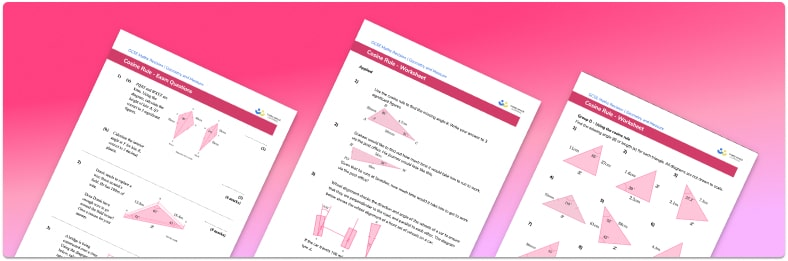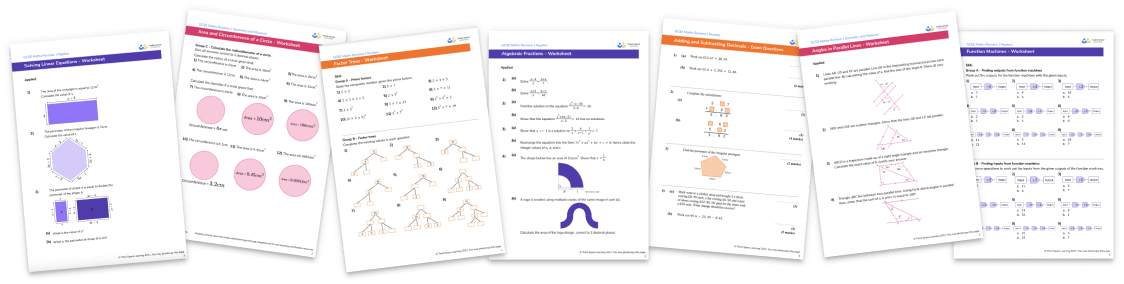# Sine And Cosine Rule Worksheet• Section 1 of the cosine worksheet contains 36 skills-based sine and cosine rule questions, in 4 groups to support differentiation
• Section 2 contains 3 applied sine and cosine rule questions with a mix of worded problems, deeper problem solving questions and GCSE higher level exam style questions
• Answers and a mark scheme for all questions are provided
• Questions follow variation theory with plenty of opportunities for students to work independently at their own level
• All questions created by fully qualified expert secondary maths teachers
• Suitable for GCSE maths revision for AQA, OCR and Edexcel exam boards

• This field is for validation purposes and should be left unchanged.

You can unsubscribe at any time (each email we send will contain an easy way to unsubscribe). To find out more about how we use your data, see our privacy policy.

### Cosine rule at a glance

The cosine rule is the relationship between one angle within a triangle and its three sides. The cosine rule states that a²=b²+c²-2bc cos(A) where a, b and c are the lengths of the sides of the triangle and A is the angle opposite side a.

The cosine rule can be used to work out missing side lengths and missing angles in non right-angled triangles.

To find the missing information, we put the values we know into the cosine rule and then solve the equation to find the missing length. When working out a missing angle we will need to use the inverse trigonometric function cos-1.

Be sure not to round your answers too early in your calculation when using the cosine rule. Also pay close attention to the specified number of decimal places or significant figures in your answer.

Looking forward, students can then progress to additional geometry worksheets, for example a 3D shapes worksheet or .For more teaching and learning support on Geometry our GCSE maths lessons provide step by step support for all GCSE maths concepts.

## Do you have KS4 students who need more focused attention to succeed at GCSE?There will be students in your class who require individual attention to help them succeed in their maths GCSEs. In a class of 30, it’s not always easy to provide.

Help your students feel confident with exam-style questions and the strategies they’ll need to answer them correctly with our dedicated GCSE maths revision programme.

Lessons are selected to provide support where each student needs it most, and specially-trained GCSE maths tutors adapt the pitch and pace of each lesson. This ensures a personalised revision programme that raises grades and boosts confidence.

Find out more